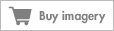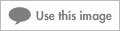Look up an image:

#### solidsGeometric shapes in three dimensions that are delimited by surfaces.See solids in : french | spanish

#### helixVolume or solid of spiral shape that turns toward the left at a constant angle.

#### cylinderVolume or solid generated by the rotation of a straight line (generatrix) moving along a curved line (directrix).

#### regular octahedronVolume or solid with eight triangular sides of equal area; it has six vertices and 12 edges.

#### parallelepipedVolume or solid with six sides (parallelograms) that are parallel in pairs.

#### cubeVolume or solid with six square sides of equal area and six equal edges; it has eight vertices.

#### pyramidVolume or solid generated by straight lines (edges) connecting the angles of a polygon (base) to the vertex and whose sides form triangles.

#### coneVolume or solid generated by the rotation of a straight line (generatrix) along a circular line (directrix) from a fixed point (vertex).

#### torusVolume or solid generated by the rotation of a circle at an equal distance from its center of rotation.

#### sphereVolume with all the points on its surface the same distance from its center; the solid thus delimited is a round ball.

#### hemisphereHalf sphere cut along its diameter.